# Python | Pandas TimedeltaIndex.intersection

Python is a great language for doing data analysis, primarily because of the fantastic ecosystem of data-centric python packages. Pandas is one of those packages and makes importing and analyzing data much easier.

Pandas` TimedeltaIndex.intersection()` function return a new Index with elements from the index that are common to both the indexes. This is a specialized intersection for TimedeltaIndex objects. It may be much faster than Index.intersection.

Syntax : TimedeltaIndex.intersection(other)

Parameters :
other : TimedeltaIndex or array-like

Return : Index or TimedeltaIndex

Example #1: Use `TimedeltaIndex.intersection()` function to find the intersection of two TimedeltaIndex objects.

 `# importing pandas as pd ` `import` `pandas as pd ` ` `  `# Create the first TimedeltaIndex object ` `tidx1 ``=` `pd.TimedeltaIndex(start ``=``'11 days 22:11:12.001124'``, periods ``=` `5``, ` `                                          ``freq ``=``'T'``, name ``=``'New_object'``) ` ` `  `# Create the seccond TimedeltaIndex object ` `tidx2 ``=` `pd.TimedeltaIndex(start ``=``'11 days 22:14:12.001124'``, periods ``=` `5``, ` `                                          ``freq ``=``'T'``, name ``=``'New_object'``) ` ` `  `# Print the first and second TimedeltaIndex object ` `print``(tidx1, ``'\n'``, tidx2) `

Output :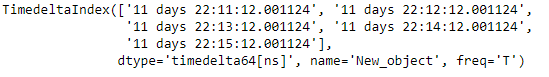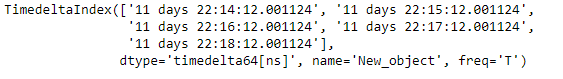Now we will use the `TimedeltaIndex.intersection()` function to find the intersection of the two objects

 `# find the intersection ` `tidx1.intersection(tidx2) `

Output :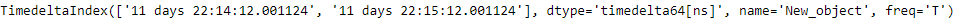As we can see in the output, the `TimedeltaIndex.intersection()` function has returned an object which contains only those elements which are common to both tidx1 and tidx2.

Example #2: Use `TimedeltaIndex.intersection()` function to find the intersection of two TimedeltaIndex objects.

 `# importing pandas as pd ` `import` `pandas as pd ` ` `  `# Create the first TimedeltaIndex object ` `tidx1 ``=` `pd.TimedeltaIndex(start ``=``'1 days 02:00:12.001124'``, ` `                    ``periods ``=` `5``, freq ``=``'D'``, name ``=``'Koala'``) ` ` `  `# Create the second TimedeltaIndex object ` `tidx2 ``=` `pd.TimedeltaIndex(start ``=``'3 days 02:00:12.001124'``,  ` `                    ``periods ``=` `5``, freq ``=``'D'``, name ``=``'Koala'``) ` ` `  `# Print the first and second TimedeltaIndex object ` `print``(tidx1, ``'\n'``, tidx2) `

Output :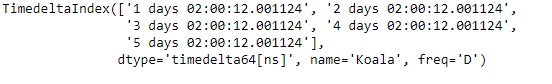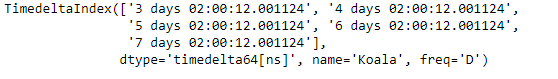Now we will use the `TimedeltaIndex.intersection()` function to find the intersection of the two objects

 `# find the intersection ` `tidx1.intersection(tidx2) `

Output :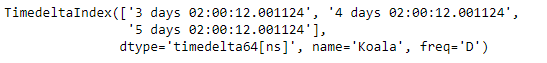As we can see in the output, the `TimedeltaIndex.intersection()` function has returned an object which contains only those elements which are common to both tidx1 and tidx2.

My Personal Notes arrow_drop_upCheck out this Author's contributed articles.

If you like GeeksforGeeks and would like to contribute, you can also write an article using contribute.geeksforgeeks.org or mail your article to contribute@geeksforgeeks.org. See your article appearing on the GeeksforGeeks main page and help other Geeks.

Please Improve this article if you find anything incorrect by clicking on the "Improve Article" button below.

Article Tags :

Be the First to upvote.

Please write to us at contribute@geeksforgeeks.org to report any issue with the above content.